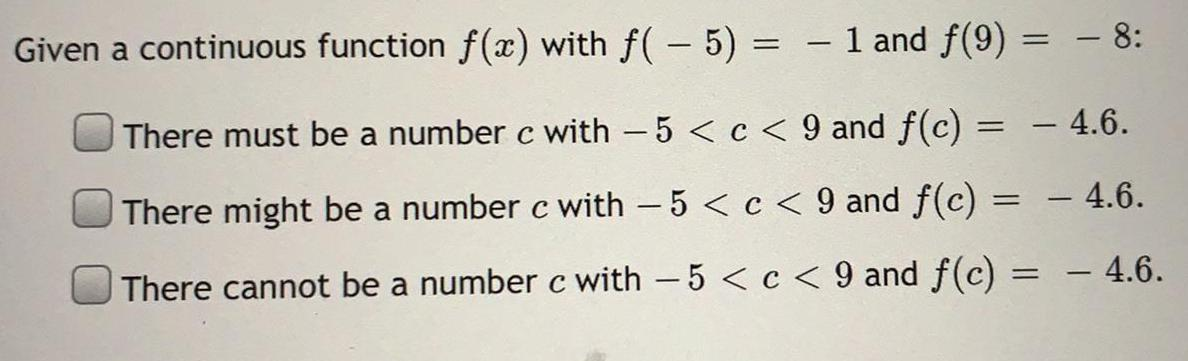Question:

# Given a continuous function f(x) with f(-5) = - 1 and f(9) =

Last updated: 7/21/2022Given a continuous function f(x) with f(-5) = - 1 and f(9) = - 8: There must be a number c with − 5 < c < 9 and f(c) = - 4.6. There might be a number c with - 5 < c < 9 and f(c) = - 4.6. There cannot be a number c with -5 < c < 9 and f(c) = - 4.6.